# Telangana SSC Board (TS SSC) Question Paper 2 for Class 10th Maths 2019 In PDF

## Telangana 10th Annual Exam Question Papers 2019 Maths Paper 2 with Solutions – Free Download

Telangana SSC Class 10 Maths 2019 Question Paper 2 with solutions is provided at BYJU’S in a downloadable pdf format, so that students can access them easily. Also, they can practice all the previous year Class 10 Maths question papers to get thorough with the exam pattern.

BYJU’S has provided solutions to Telangana SSC Board Question Paper 2 for Class 10th Maths 2019 to help the students in verifying their answers and also to refer whenever they need to get some assistance in order to solve them. Telangana SSC board conducts Maths exam with two papers, each having part A and part B, hence, students should be perfect in understanding the pattern of questions that appear in the exam.

### Telangana Board SSC Class 10 Maths 2019 Question Paper 2 with Solutions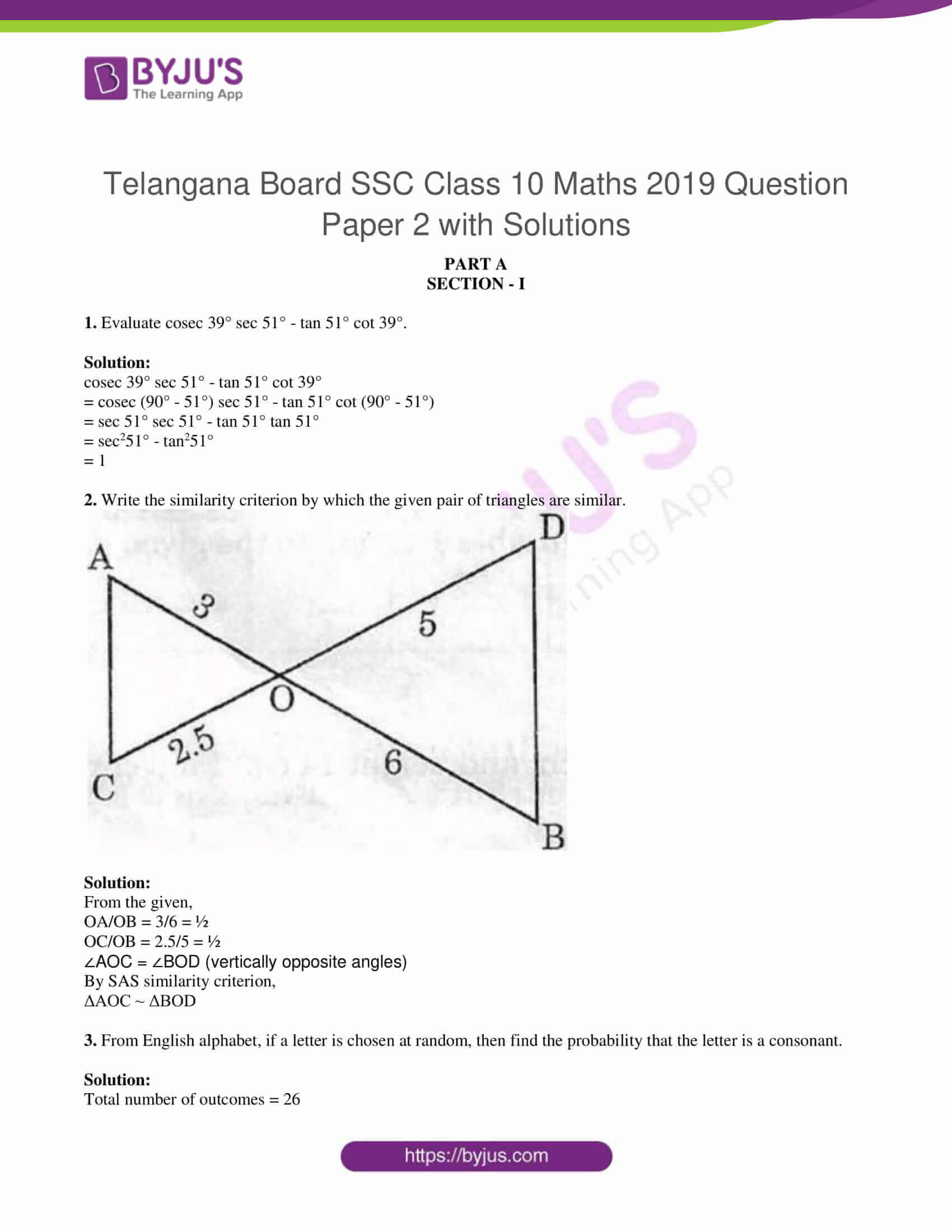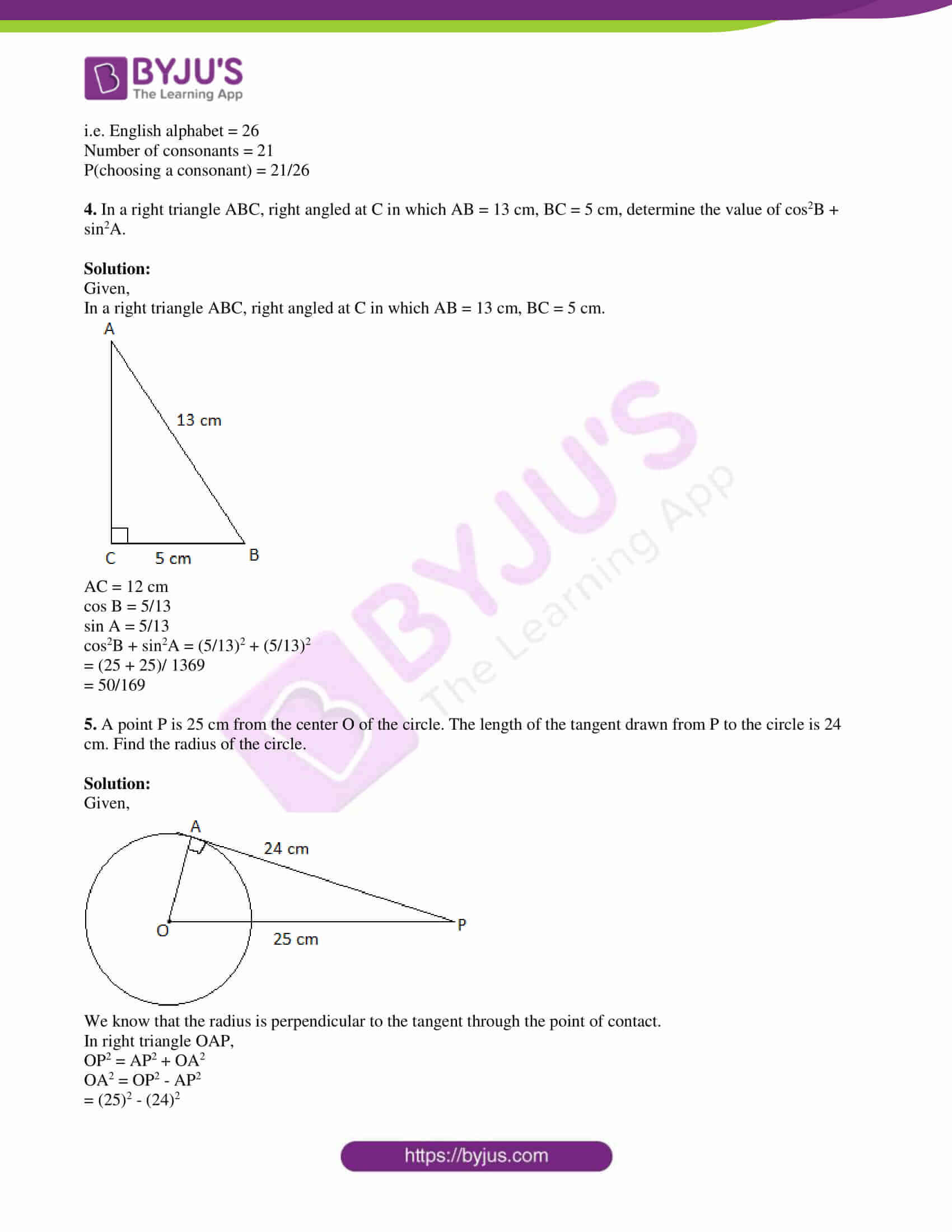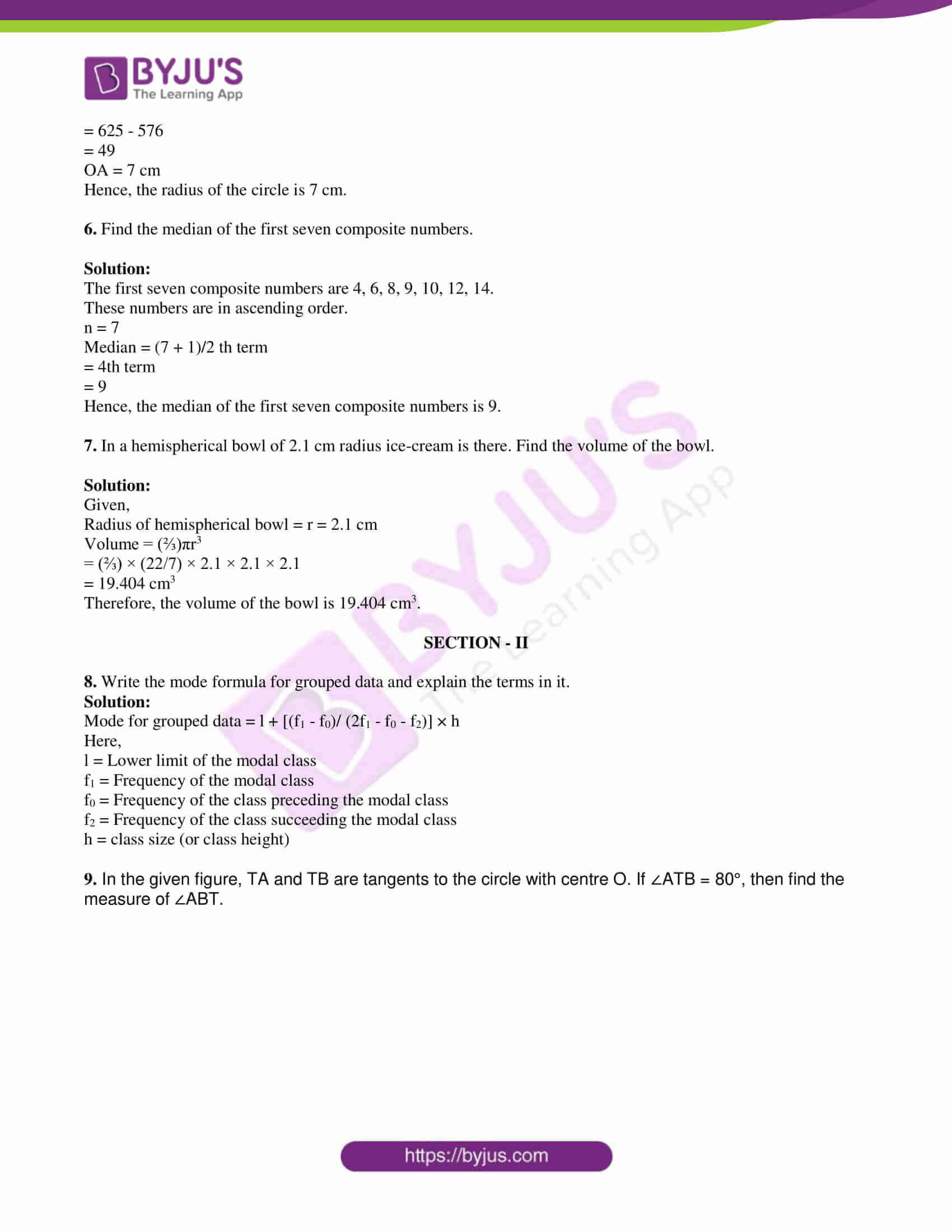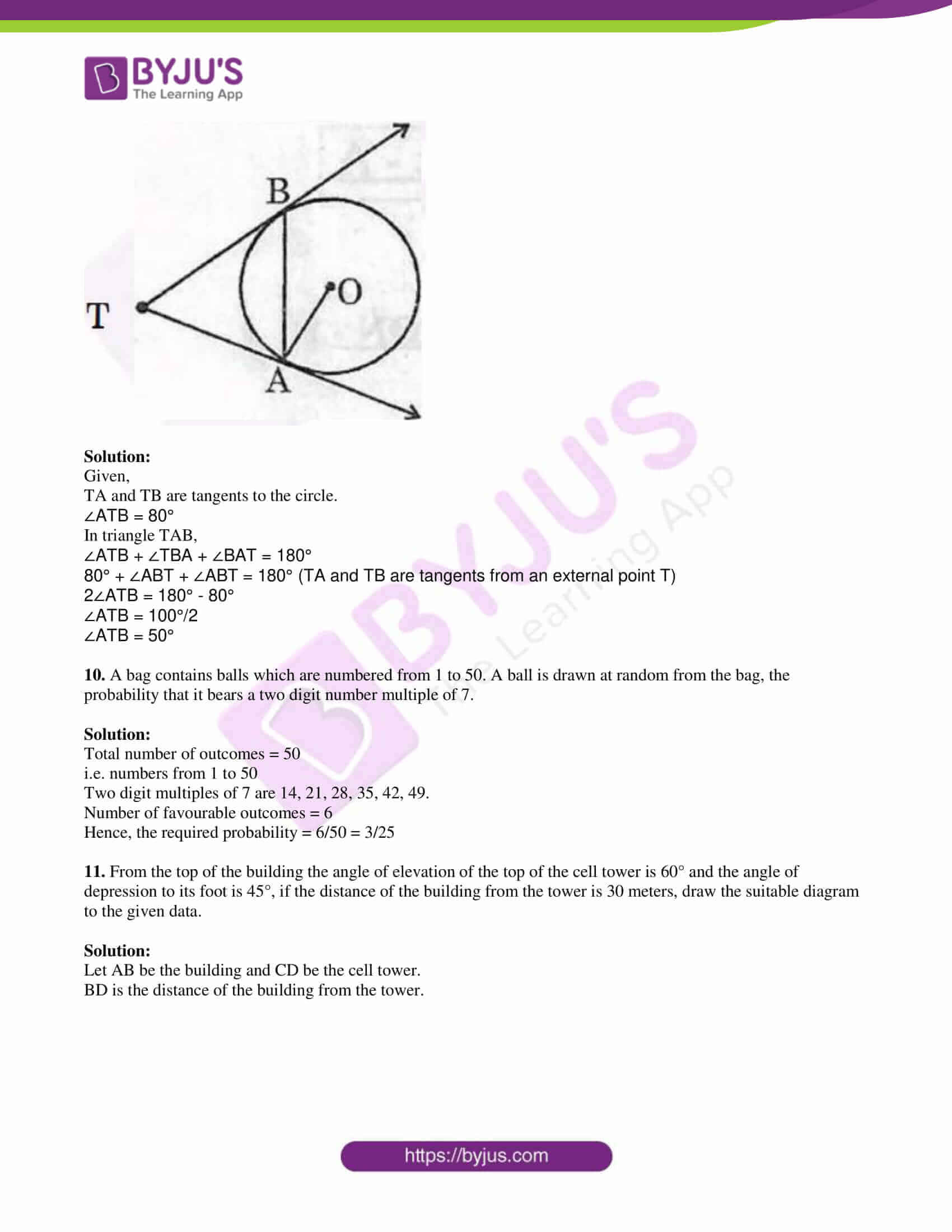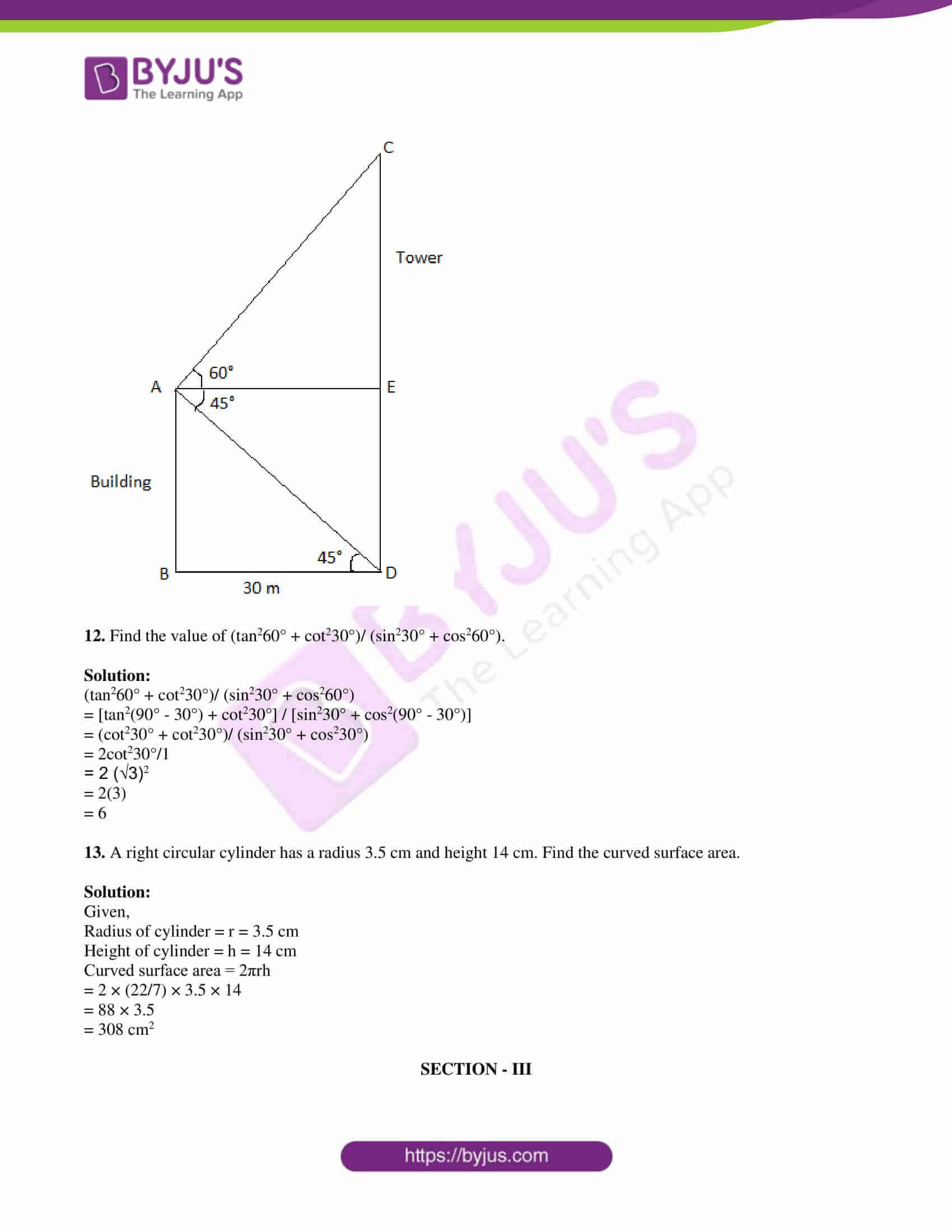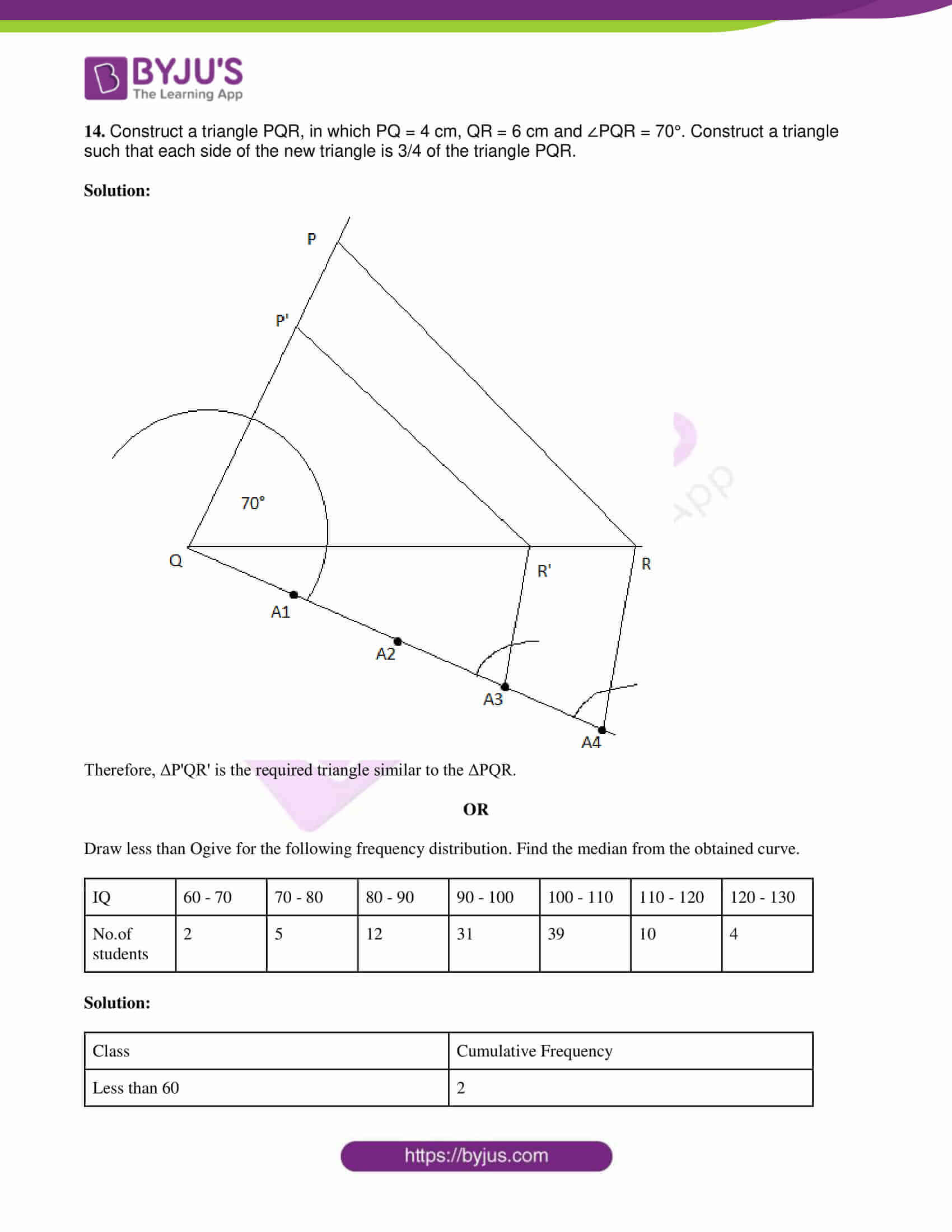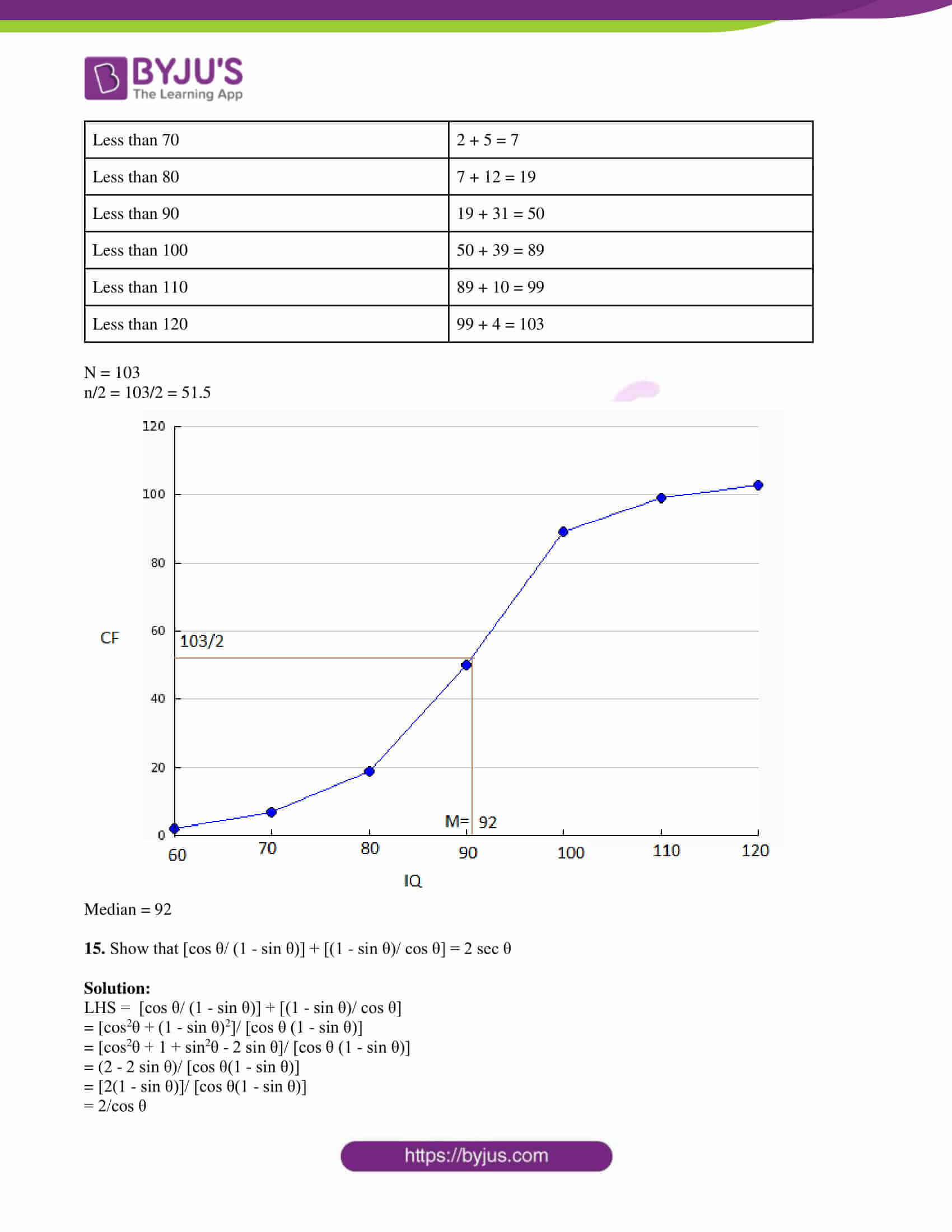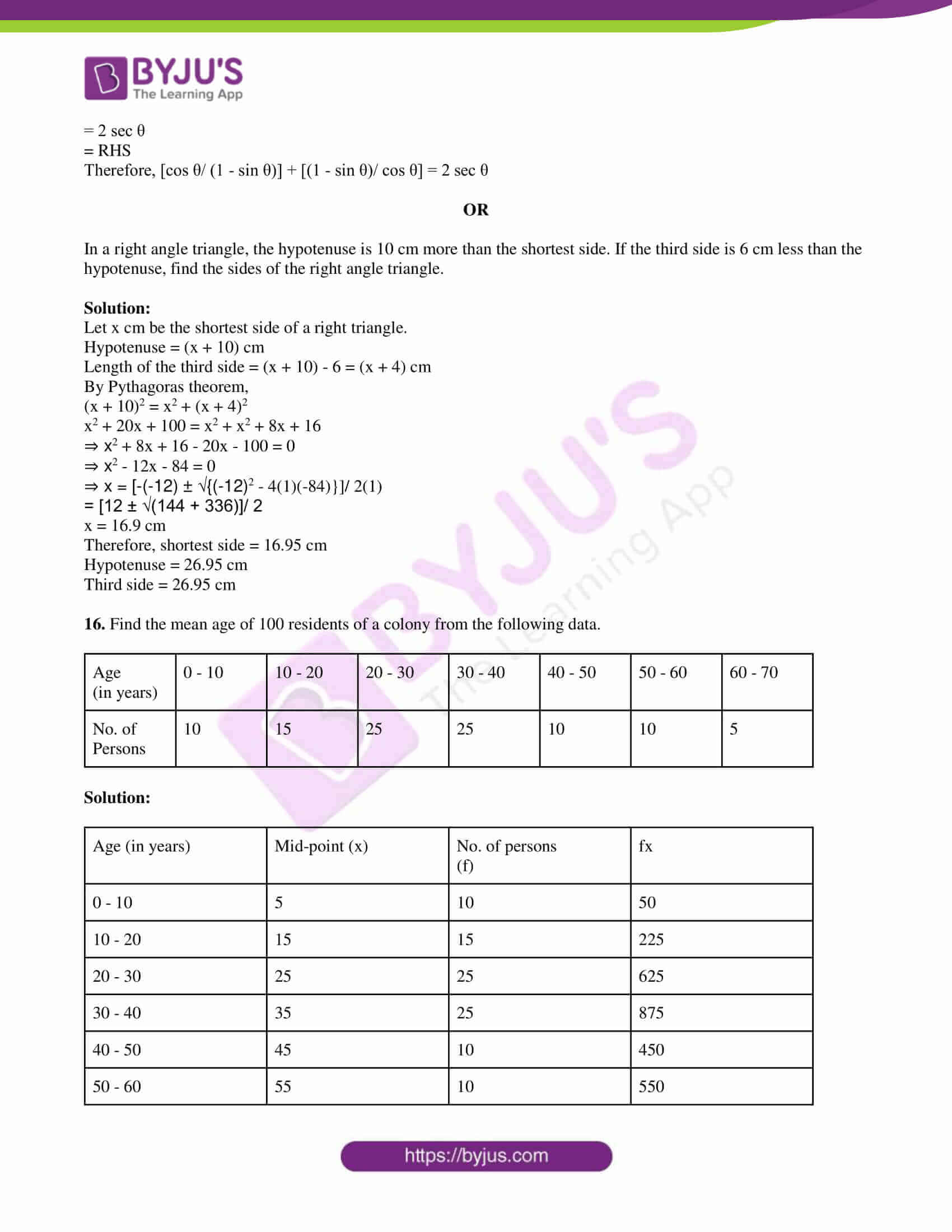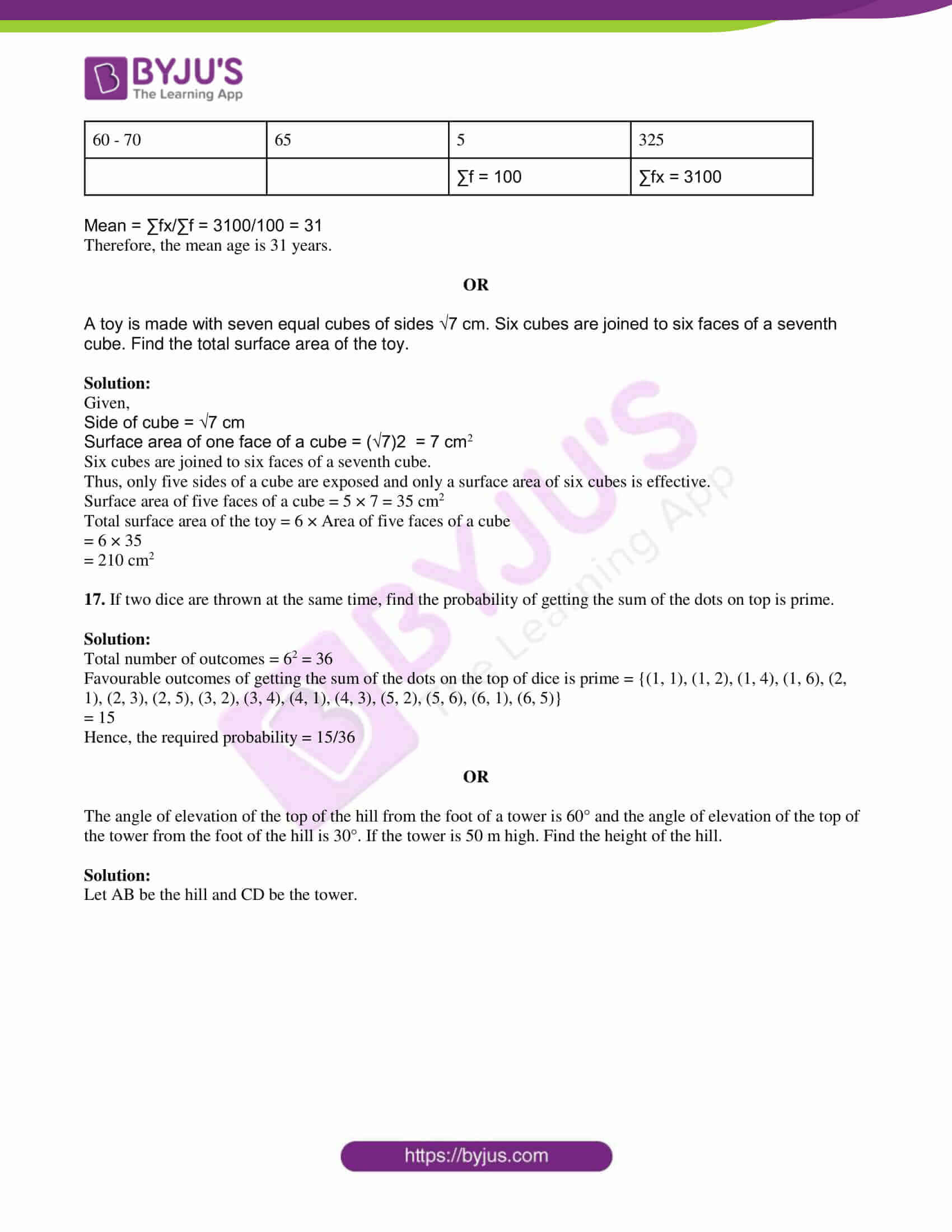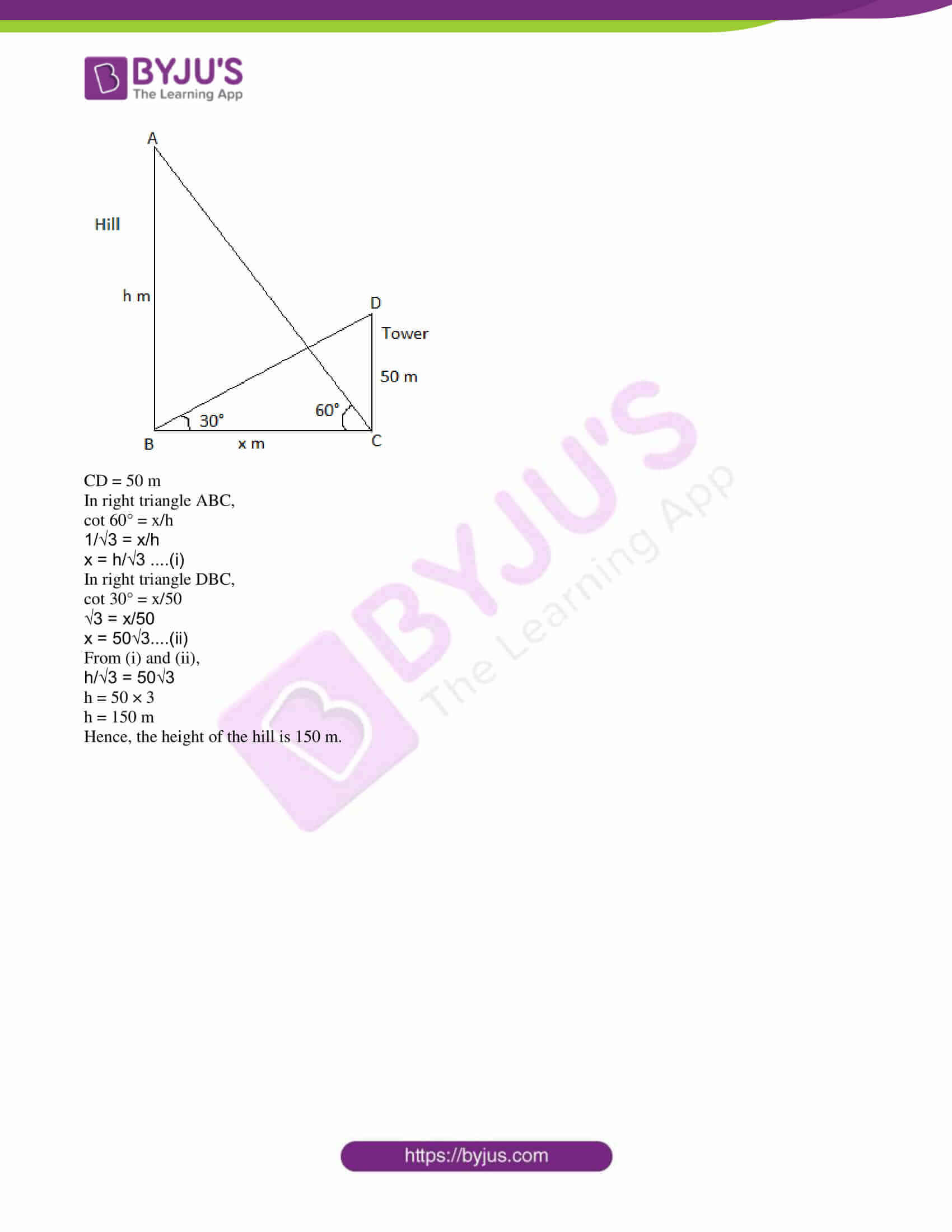PART A

SECTION – I

1. Evaluate cosec 39° sec 51° – tan 51° cot 39°.

Solution:

cosec 39° sec 51° – tan 51° cot 39°

= cosec (90° – 51°) sec 51° – tan 51° cot (90° – 51°)

= sec 51° sec 51° – tan 51° tan 51°

= sec251° – tan251°

= 1

2. Write the similarity criterion by which the given pair of triangles are similar.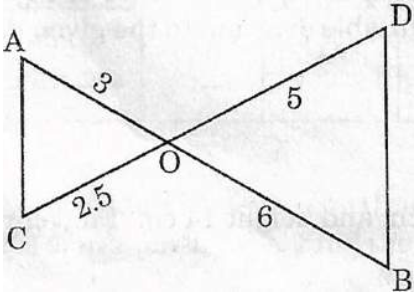Solution:

From the given,

OA/OB = 3/6 = ½

OC/OB = 2.5/5 = ½

∠AOC = ∠BOD (vertically opposite angles)

By SAS similarity criterion,

ΔAOC ~ ΔBOD

3. From English alphabet, if a letter is chosen at random, then find the probability that the letter is a consonant.

Solution:

Total number of outcomes = 26

i.e. English alphabet = 26

Number of consonants = 21

P(choosing a consonant) = 21/26

4. In a right triangle ABC, right angled at C in which AB = 13 cm, BC = 5 cm, determine the value of cos2B + sin2A.

Solution:

Given,

In a right triangle ABC, right angled at C in which AB = 13 cm, BC = 5 cm.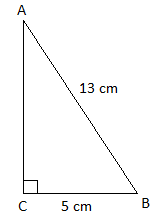AC = 12 cm

cos B = 5/13

sin A = 5/13

cos2B + sin2A = (5/13)2 + (5/13)2

= (25 + 25)/ 1369

= 50/169

5. A point P is 25 cm from the center O of the circle. The length of the tangent drawn from P to the circle is 24 cm. Find the radius of the circle.

Solution:

Given,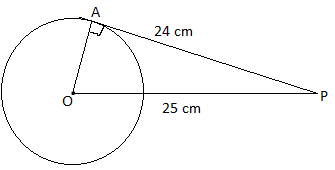We know that the radius is perpendicular to the tangent through the point of contact.

In right triangle OAP,

OP2 = AP2 + OA2

OA2 = OP2 – AP2

= (25)2 – (24)2

= 625 – 576

= 49

OA = 7 cm

Hence, the radius of the circle is 7 cm.

6. Find the median of the first seven composite numbers.

Solution:

The first seven composite numbers are 4, 6, 8, 9, 10, 12, 14.

These numbers are in ascending order.

n = 7

Median = (7 + 1)/2 th term

= 4th term

= 9

Hence, the median of the first seven composite numbers is 9.

7. In a hemispherical bowl of 2.1 cm radius ice-cream is there. Find the volume of the bowl.

Solution:

Given,

Radius of hemispherical bowl = r = 2.1 cm

Volume = (⅔)πr3

= (⅔) × (22/7) × 2.1 × 2.1 × 2.1

= 19.404 cm3

Therefore, the volume of the bowl is 19.404 cm3.

SECTION – II

8. Write the mode formula for grouped data and explain the terms in it.

Solution:

Mode for grouped data = l + [(f1 – f0)/ (2f1 – f0 – f2)] × h

Here,

l = Lower limit of the modal class

f1 = Frequency of the modal class

f0 = Frequency of the class preceding the modal class

f2 = Frequency of the class succeeding the modal class

h = class size (or class height)

9. In the given figure, TA and TB are tangents to the circle with centre O. If ∠ATB = 80°, then find the measure of ∠ABT.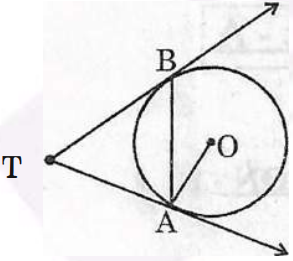Solution:

Given,

TA and TB are tangents to the circle.

∠ATB = 80°

In triangle TAB,

∠ATB + ∠TBA + ∠BAT = 180°

80° + ∠ABT + ∠ABT = 180° (TA and TB are tangents from an external point T)

2∠ATB = 180° – 80°

∠ATB = 100°/2

∠ATB = 50°

10. A bag contains balls which are numbered from 1 to 50. A ball is drawn at random from the bag, the probability that it bears a two digit number multiple of 7.

Solution:

Total number of outcomes = 50

i.e. numbers from 1 to 50

Two digit multiples of 7 are 14, 21, 28, 35, 42, 49.

Number of favourable outcomes = 6

Hence, the required probability = 6/50 = 3/25

11. From the top of the building the angle of elevation of the top of the cell tower is 60° and the angle of depression to its foot is 45°, if the distance of the building from the tower is 30 meters, draw the suitable diagram to the given data.

Solution:

Let AB be the building and CD be the cell tower.

BD is the distance of the building from the tower.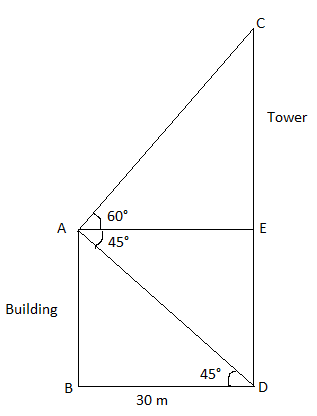12. Find the value of (tan260° + cot230°)/ (sin230° + cos260°).

Solution:

(tan260° + cot230°)/ (sin230° + cos260°)

= [tan2(90° – 30°) + cot230°] / [sin230° + cos2(90° – 30°)]

= (cot230° + cot230°)/ (sin230° + cos230°)

= 2cot230°/1

= 2 (√3)2

= 2(3)

= 6

13. A right circular cylinder has a radius 3.5 cm and height 14 cm. Find the curved surface area.

Solution:

Given,

Radius of cylinder = r = 3.5 cm

Height of cylinder = h = 14 cm

Curved surface area = 2πrh

= 2 × (22/7) × 3.5 × 14

= 88 × 3.5

= 308 cm2

SECTION – III

14. Construct a triangle PQR, in which PQ = 4 cm, QR = 6 cm and ∠PQR = 70°. Construct a triangle such that each side of the new triangle is 3/4 of the triangle PQR.

Solution: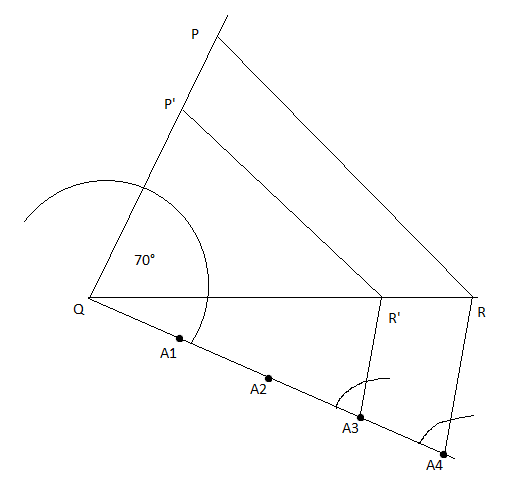Therefore, ΔP’QR’ is the required triangle similar to the ΔPQR.

OR

Draw less than Ogive for the following frequency distribution. Find the median from the obtained curve.

 IQ 60 – 70 70 – 80 80 – 90 90 – 100 100 – 110 110 – 120 120 – 130 No.of students 2 5 12 31 39 10 4

Solution:

 Class Cumulative Frequency Less than 60 2 Less than 70 2 + 5 = 7 Less than 80 7 + 12 = 19 Less than 90 19 + 31 = 50 Less than 100 50 + 39 = 89 Less than 110 89 + 10 = 99 Less than 120 99 + 4 = 103

N = 103

n/2 = 103/2 = 51.5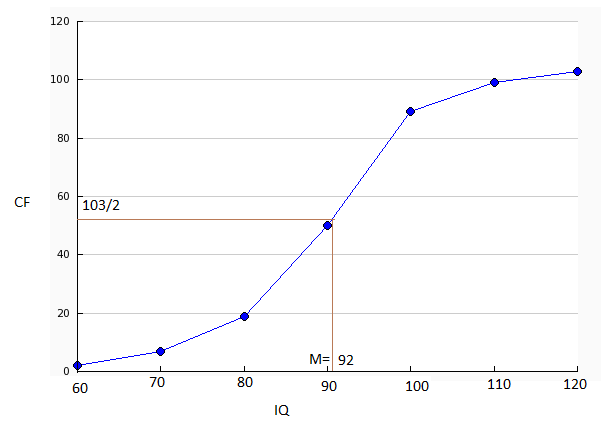Median = 92

15. Show that [cos θ/ (1 – sin θ)] + [(1 – sin θ)/ cos θ] = 2 sec θ

Solution:

LHS = [cos θ/ (1 – sin θ)] + [(1 – sin θ)/ cos θ]

= [cos2θ + (1 – sin θ)2]/ [cos θ (1 – sin θ)]

= [cos2θ + 1 + sin2θ – 2 sin θ]/ [cos θ (1 – sin θ)]

= (2 – 2 sin θ)/ [cos θ(1 – sin θ)]

= [2(1 – sin θ)]/ [cos θ(1 – sin θ)]

= 2/cos θ

= 2 sec θ

= RHS

Therefore, [cos θ/ (1 – sin θ)] + [(1 – sin θ)/ cos θ] = 2 sec θ

OR

In a right angle triangle, the hypotenuse is 10 cm more than the shortest side. If the third side is 6 cm less than the hypotenuse, find the sides of the right angle triangle.

Solution:

Let x cm be the shortest side of a right triangle.

Hypotenuse = (x + 10) cm

Length of the third side = (x + 10) – 6 = (x + 4) cm

By Pythagoras theorem,

(x + 10)2 = x2 + (x + 4)2

x2 + 20x + 100 = x2 + x2 + 8x + 16

⇒ x2 + 8x + 16 – 20x – 100 = 0

⇒ x2 – 12x – 84 = 0

⇒ x = [-(-12) ± √{(-12)2 – 4(1)(-84)}]/ 2(1)

= [12 ± √(144 + 336)]/ 2

x = 16.9 cm

Therefore, shortest side = 16.95 cm

Hypotenuse = 26.95 cm

Third side = 26.95 cm

16. Find the mean age of 100 residents of a colony from the following data.

 Age (in years) 0 – 10 10 – 20 20 – 30 30 – 40 40 – 50 50 – 60 60 – 70 No. of Persons 10 15 25 25 10 10 5

Solution:

 Age (in years) Mid-point (x) No. of persons (f) fx 0 – 10 5 10 50 10 – 20 15 15 225 20 – 30 25 25 625 30 – 40 35 25 875 40 – 50 45 10 450 50 – 60 55 10 550 60 – 70 65 5 325 ∑f = 100 ∑fx = 3100

Mean = ∑fx/∑f = 3100/100 = 31

Therefore, the mean age is 31 years.

OR

A toy is made with seven equal cubes of sides √7 cm. Six cubes are joined to six faces of a seventh cube. Find the total surface area of the toy.

Solution:

Given,

Side of cube = √7 cm

Surface area of one face of a cube = (√7)2 = 7 cm2

Six cubes are joined to six faces of a seventh cube.

Thus, only five sides of a cube are exposed and only a surface area of six cubes is effective.

Surface area of five faces of a cube = 5 × 7 = 35 cm2

Total surface area of the toy = 6 × Area of five faces of a cube

= 6 × 35

= 210 cm2

17. If two dice are thrown at the same time, find the probability of getting the sum of the dots on top is prime.

Solution:

Total number of outcomes = 62 = 36

Favourable outcomes of getting the sum of the dots on the top of dice is prime = {(1, 1), (1, 2), (1, 4), (1, 6), (2, 1), (2, 3), (2, 5), (3, 2), (3, 4), (4, 1), (4, 3), (5, 2), (5, 6), (6, 1), (6, 5)}

= 15

Hence, the required probability = 15/36

OR

The angle of elevation of the top of the hill from the foot of a tower is 60° and the angle of elevation of the top of the tower from the foot of the hill is 30°. If the tower is 50 m high. Find the height of the hill.

Solution:

Let AB be the hill and CD be the tower.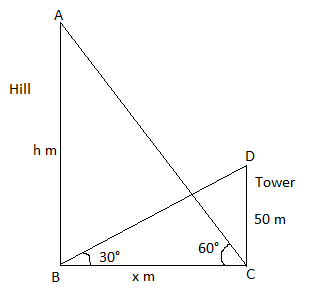CD = 50 m

In right triangle ABC,

cot 60° = x/h

1/√3 = x/h

x = h/√3 ….(i)

In right triangle DBC,

cot 30° = x/50

√3 = x/50

x = 50√3….(ii)

From (i) and (ii),

h/√3 = 50√3

h = 50 × 3

h = 150 m

Hence, the height of the hill is 150 m.